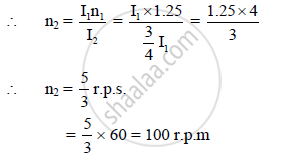HSC Science (Electronics) 12th Board ExamMaharashtra State Board
Share

# A Ballet Dancer Spins About a Vertical Axis at 2.5\u0001 Rad/S with His Both Arms Outstretched. with the Arms Folded, the Moment of Inertia About the Same Axis of Rotation Changes by 25%. Calculate the New Speed of Rotation in R.P.M - HSC Science (Electronics) 12th Board Exam - Physics

ConceptPhysical Significance of M.I (Moment of Inertia)

#### Question

A ballet dancer spins about a vertical axis at 2.5Π rad/s with his both arms outstretched. With the arms folded, the moment of inertia about the same axis of rotation changes by 25%. Calculate the new speed of rotation in r.p.m.

#### Solution

ω1 = 2.5 π rad/s = 2πn1

∴ I_2 = 3/4 I_1,n_2 (r.p.m) = ?

By conservation of angular momentum,

I2ω2 = I1ω1 or I2 (2πn2) = I1 (2πn1)The new speed of rotation in r.p.m is 100 r.p.m

Is there an error in this question or solution?

#### APPEARS IN

2012-2013 (October) (with solutions)
Question 1.1 | 7.00 marks
Solution A Ballet Dancer Spins About a Vertical Axis at 2.5\u0001 Rad/S with His Both Arms Outstretched. with the Arms Folded, the Moment of Inertia About the Same Axis of Rotation Changes by 25%. Calculate the New Speed of Rotation in R.P.M Concept: Physical Significance of M.I (Moment of Inertia).
S